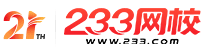#计算机二级

•扫描二维码进入

• 题 库扫描二维码进入

• 移动233网校移动

• 移动APP扫描二维码下载APP

## 2019年3月计算机二级MySQL强化练习及答案(12)

1.下列程序的输出结果是( )。

#include

int b=2：

int func(int*a)

{ b+=*a;return b;}

main()

{ int a=1，t=2;

t+=func(&a)：

printf("%d\n"，t);

}

A.4

B.5

C.6

D.8

2.若有定义“float a=25，b，*p=&b;”，则下列对赋值语句“*p=a;”和“p=&a;”的正确解释为( )。

A.两个语句都是将变量a的值赋予变量P

B.*p=a是使P指向变量a，而p=&a是将变量的值赋予变量指针P

c.*p=a是将变量a的值赋予变量b，而&a是使P指向变量a

D.两个语句都是使P指向变量a

3.对两个数组a和b进行下列初始化：

char m[]="1234567";

char n[]={'1'，'2'，'3'，'4'，'5'，'6'，'7'};

A.数组m与数组n完全相同

B.数组m与数组n长度相同

C.数组m比数组n长1

D.数组m与数组n中都存放字符串

4.下列程序输出结果是( )。

#include

main()

{ char a[]={'a'，'b'，'C'，'d'，'e'，'f'，'\0').

int i，j;

i=sizeof(a);

j=strlen(a);

printf("%d，%d"，i，j);

}

A.7，7

B.7，6

C.6，6

D.6，7

5.软件生命周期是指( )。

A.软件产品从提出、实现、使用维护到停止使用退役的过程

B.软件从需求分析、设计、实现到测试完成的过程

C.软件的开发过程

D.软件的运行维护过程

6.下列程序的运行结果是( )。

#include

main()

{ int m=1，n=2，k=3;

printf("s=%d"，s);

}

A.sum=18

B.sum=10

C.sum=9

D.sum=25

7.有以下程序：

fun(int x，int y){return(x+y);)

main()

{ int a=1，b=2，c=2，sum;

sum=fun((a++，b++，a+b)，c++);

printf("%d\n"，sum);

}

A.6

B.7

C.8

D.9

8.设x=011050，则x=x&01252的值是( )。

A.0000001000101000

B.1111110100011001

C.0000001011100010

D.1100000000101000

9.有以下程序：

int fun(int x)

{ int p;

if(x==0||x==1)return(3);

p=x-fun(x-2);

return p;

}

main()

{ printf("%d\n"，fun(7));}

A.7

B.3

C.2

D.0

A.存放读入数据项的存储区

B.一个指向所读文件的文件指针

C.一个指针，是指向输入数据存放在内存中的起始地址

D.一个整型变量，代表要读入的数据项总数

• 海量免费模拟试题，在线测试。

• 各科每年真题试卷，实战演练！

• 逐章逐节针对练习，专项突破。

• 每天10道最新试题，随机练习。

• QQ群号：806931213

扫描二维码加群• ##### 微信订阅号：kszx233

扫描二维码加群